Next: 8.2 Continuity Up: 8. Continuity Previous: 8. Continuity   Index

# 8.1 Compositions with Sequences

8.1   Definition (Composition) Let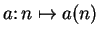be a complex sequence. Let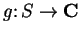be a function such that, and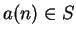for all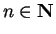. Then the composition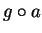is the sequence such that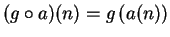for all. If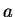is a sequence, I will often write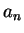instead of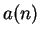. Then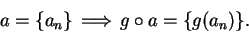8.2   Examples. Ifandfor all, then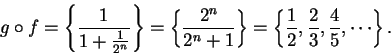Figure a) below shows representations of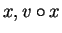and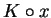where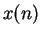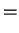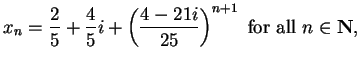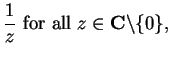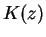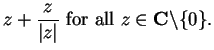(8.3)

I leave it to you to check that, and, and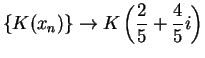. Figure b) shows representations forand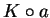whereand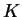is defined as in (8.3). Here it is easy to check that. From the figure,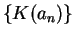doesn't appear to converge.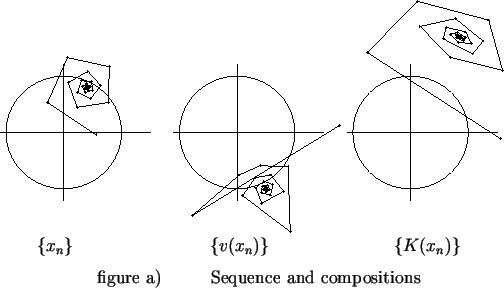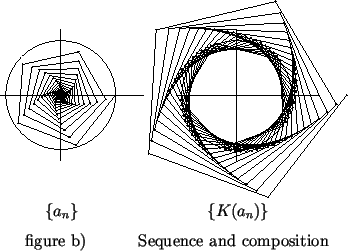8.4   Exercise. A Let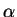be a non-zero complex number with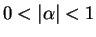. Let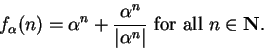Under what conditions ondoesconverge? What does it converge to? (Your answer should show that the sequencefrom the previous example does not converge.)

8.5   Definition (Complex function.) By a complex function I will mean a function whose domain is a subset of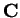, and whose codomain is. I will consider functions from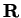toto be complex functions by identifying a function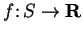with a function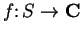in the expected manner.Next: 8.2 Continuity Up: 8. Continuity Previous: 8. Continuity   Index